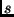Next: Transport-level Protocols Up: Coordination Protocol (CP) Previous: Detecting Network Delay and

## Calculating Loss Rate and Bandwidth Availability

Calculation of loss rate and bandwidth availability make use of equation-based congestion control methods described in Floyd et al. in their work on TCP-friendly rate control (TFRC) .

Loss rate, a central input parameter into the bandwidth availability equation, is calculated using a loss history and loss events rather than individual packet losses. By using a loss event rate rather than a simple lost packet rate, we provide a more stable handling of lost packet bursts. The reader is referred to  for more details.

Calculation of available bandwidth makes use of the equation:(1)

whereis the transmit rate (bytes/sec),is the packet size (bytes),is the round trip time (sec),is the loss event rate on the interval [0,1.0],is the TCP retransmission timeout (sec), andis the number of packets acknowledged by a single TCP acknowledgement.

The resulting quantity, which we refer to as current bandwidth availability, is calculated at the remote AP, and then passed using the CP header to each endpoint in the cluster. Similarly, the event loss rate is also passed on to endpoints to inform them of current network conditions.

We emphasize here that the use of the above equation to calculate bandwidth availability for the cluster application makes the aggregate data flow from one AP to another TCP-compatible.Next: Transport-level Protocols Up: Coordination Protocol (CP) Previous: Detecting Network Delay and
David Ott 2002-04-16